Homework Help Question & Answers

# In mathematical language, Newton's Law of Gravity expresses the force of gravity, F, between two objects in the following way: F= (GMm)/r^2 where M is the mass of one object, m is the mass of the other object, r is the distance between the two objects

In mathematical language, Newton's Law of Gravity expresses the force of gravity, F, between two objects in the following way:

F= (GMm)/r^2

where M is the mass of one object, m is the mass of the other object, r is the distance between the two objects, and G is the gravitational constant. Which of the following statements best describes what this formula says?

a)The force of gravity between two objects depends on the product of their masses and the distance between them.

b)The force of gravity between two objects depends on the sum of their masses and the distance between them.

c)The force of gravity between two objects depends on the product of their masses and the inverse square of the distance between them.

I would say c. Is this right? Thanks

#### Homework Answers

Answer #1
yeah
answered by: CRESELLE ROSE
Know the answer?
Your Answer:

#### Post as a guest

Your Name:

What's your source?

#### Earn Coin

Coins can be redeemed for fabulous gifts.

Not the answer you're looking for? Ask your own homework help question. Our experts will answer your question WITHIN MINUTES for Free.
Similar Homework Help Questions
• ### FERENCES MAILINGS REVIEWVIEWFoxit PDF The force of gravity, F, exerted between two objects is equal to...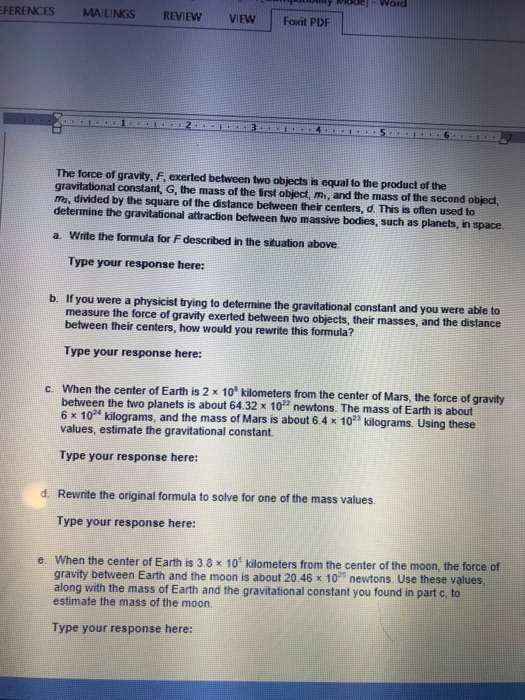FERENCES MAILINGS REVIEWVIEWFoxit PDF The force of gravity, F, exerted between two objects is equal to the product of the gravitational constant, G, the mass of the irst object, m, and the mass of the second object, ma, divided by the square of the distance between their centers, d. This is often used to determine the gravitational attraction behween two massive bodies, such as planets, in space. a. Write the formula for F described in the situation above. Type your...

• ### Given Newton's law of universal gravitation where F is the force between two masses objects, m1...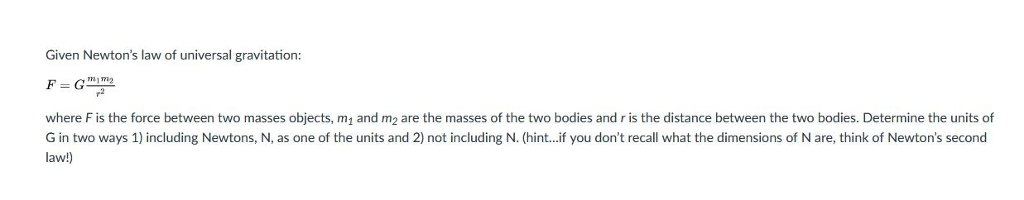Given Newton's law of universal gravitation where F is the force between two masses objects, m1 and m2 are the masses of the two bodies and r is the distance between the two bodies. Determine the units of G in two ways 1) including Newtons, N, as one of the units and 2) not including N. (hint...if you don't recall what the dimensions of N are, think of Newton's second law!

• ### Two identical objects of mass m are a distance R apart and feel a gravitational force...

Two identical objects of mass m are a distance R apart and feel a gravitational force of attraction of F. If the distance increases to 5R, what will be the force between the two objects? Select one: a. F/5 b. F/25 c. F d. 25F e. 5F

• ### General Overview: Any two objects will exert an attractive force on the other. The force is...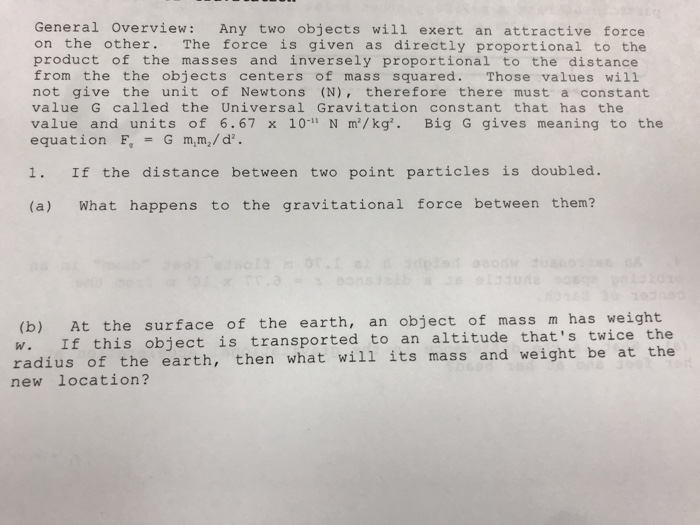General Overview: Any two objects will exert an attractive force on the other. The force is given as directly proportional to the product of the masses and inversely proportional to the distance from the the objects centers of mass squared. Those values will not give the unit of Newtons (N), therefore there must a constant value G called the Universal Gravitation constant that has the value and units of 6.67 x 10-11 N m/ kg". Big G gives meaning to...

• ### 3. (6 pts) Newton's law of gravity and Coulomb's law are both inverse-square laws. Consequently, there...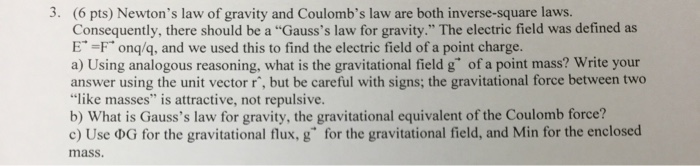3. (6 pts) Newton's law of gravity and Coulomb's law are both inverse-square laws. Consequently, there should be a "Gauss's law for gravity." The electric field was defined as E" =F" onq/q, and we used this to find the electric field of a point charge. a) Using analogous reasoning, what is the gravitational field g" of a point mass? Write your answer using the unit vector r', but be careful with signs; the gravitational force between two "like masses" is...

• ### I NEED HELP~ ACTIVITY 4 Activity 4: Newton's Law of Gravity: The force of gravity between...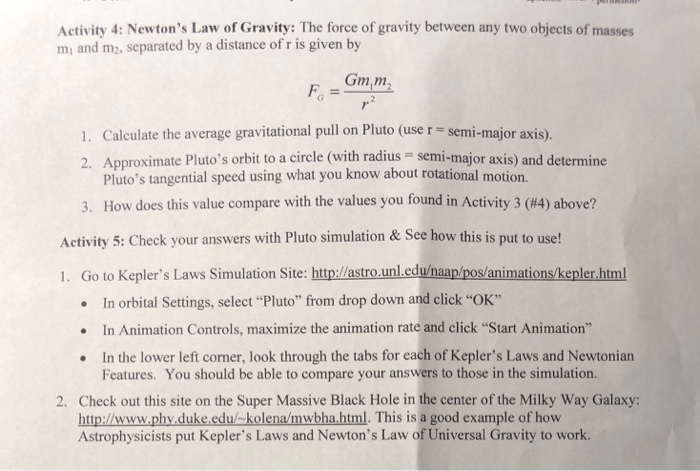I NEED HELP~ ACTIVITY 4 Activity 4: Newton's Law of Gravity: The force of gravity between any two objects of masses mi and m2, separated by a distance of r is given by Gmm 1. Calculate the average gravitational pull on Pluto (use r - semi-major axis). 2. Approximate Pluto's orbit to a circle (with radius semi-major axis) and determine Pluto's tangential speed using what you know about rotational motion. How does this value compare with the values you found...

• ### Which of the following statements are true? Because the Earth has mass it produces gravity. There...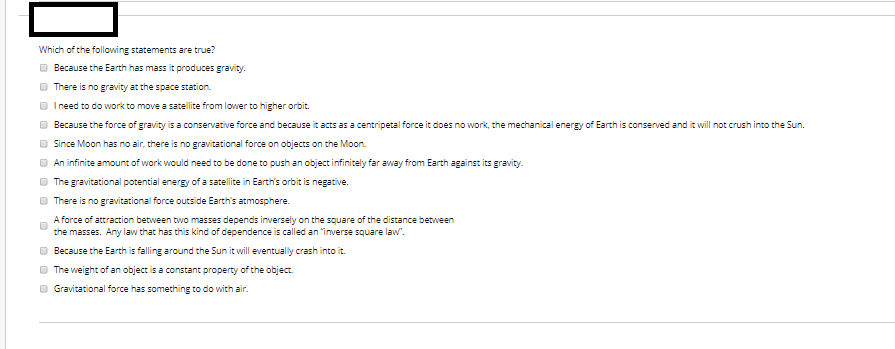Which of the following statements are true? Because the Earth has mass it produces gravity. There is no gravity at the space station I need to do work to move a satellite from lower to higher orbit. Because the force of gravity is a conservative force and because it acts as a centripetal force it does no work, the mechanical energy of Earth is conserved and it will not crush into the Sun. Since Moon has no air, there is...

• ### Use Newton's law of universal Gravitation to estimate force exerted by one object on another: F...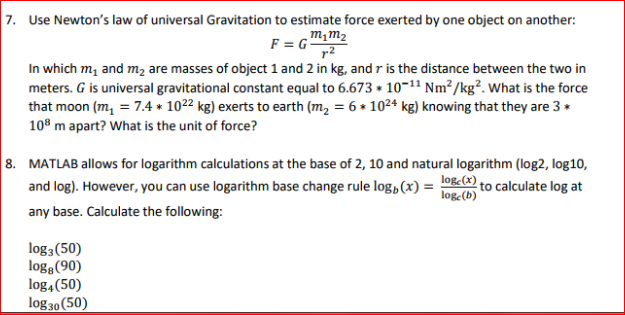Use Newton's law of universal Gravitation to estimate force exerted by one object on another: F = G m_1 m_2/r^2 In which m_1 and m_2 are masses of object 1 and 2 in kg, and r is the distance between the two in meters. G is universal gravitational constant equal to 6.673 * 10^-11 Nm ^2/kg^2. What is the force that moon (m_l = 7.4 * 10^22 kg) exerts to earth (m_2 = 6 * 10^24 kg) knowing that they...

• ### 2. According to Newton's Law of Universal Gravitation, the gravitational force on an object of ma...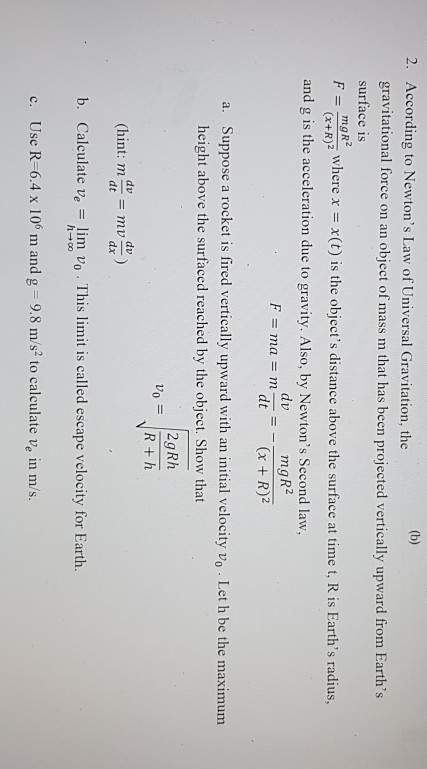Can you please give me the whole solution for this question! Thanks 2. According to Newton's Law of Universal Gravitation, the gravitational force on an object of mass m that has been projected vertically upward from Earth's surface is F( is the objer s distan boe he urfac at time t, Ris Earth's radius, ngR (x+R)2 and g is the acceleration due to gravity. Also, by Newton's Second law, mgR2 (x +R)2 dv F = mal = m dt =...

• ### Learning Goal: To understand Newton's law of gravitation and the distinction between inertial and gravitational masses....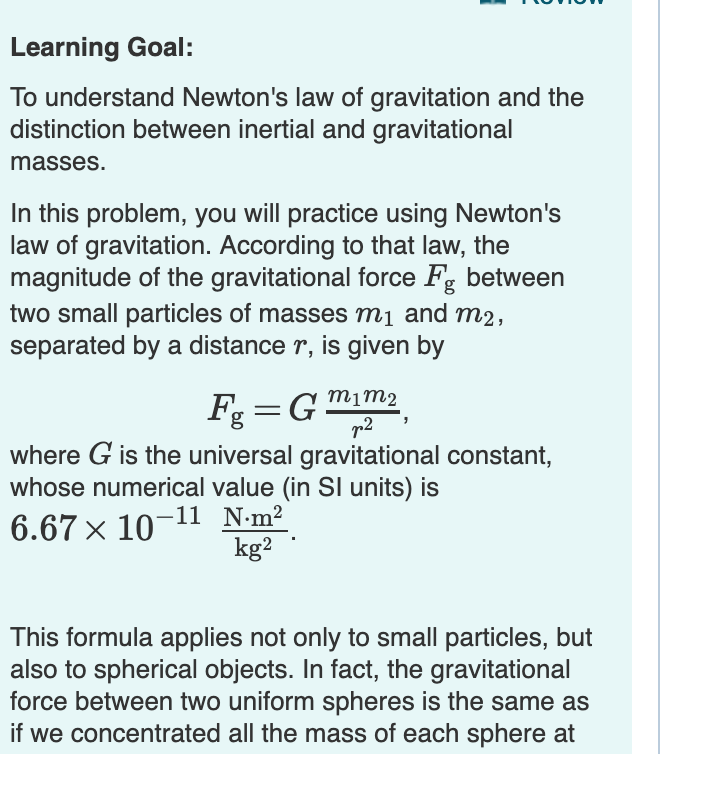Learning Goal: To understand Newton's law of gravitation and the distinction between inertial and gravitational masses. In this problem, you will practice using Newton's law of gravitation. According to that law, the magnitude of the gravitational force Fg between two small particles of masses m1 and m2 separated by a distance r, is given by m1m2 T2 where G is the universal gravitational constant, whose numerical value (in SI units) is 6.67 x 10-11 Nm2 kg2 This formula applies not...

Free Homework App

Scan Your Homework
to Get Instant Free Answers
Need Online Homework Help?

Get Answers For Free
Most questions answered within 3 hours.